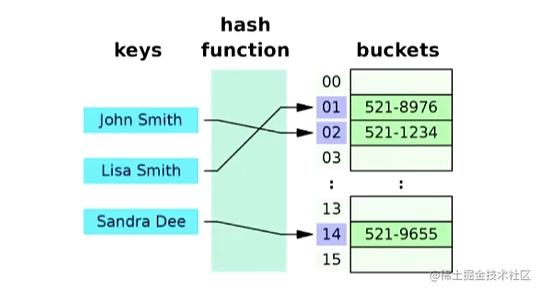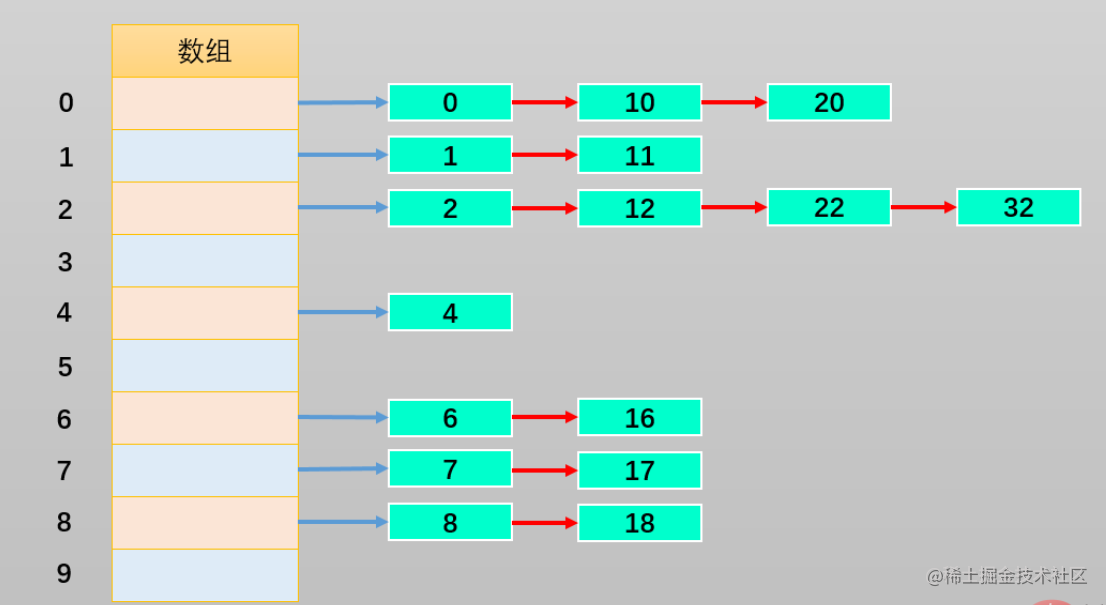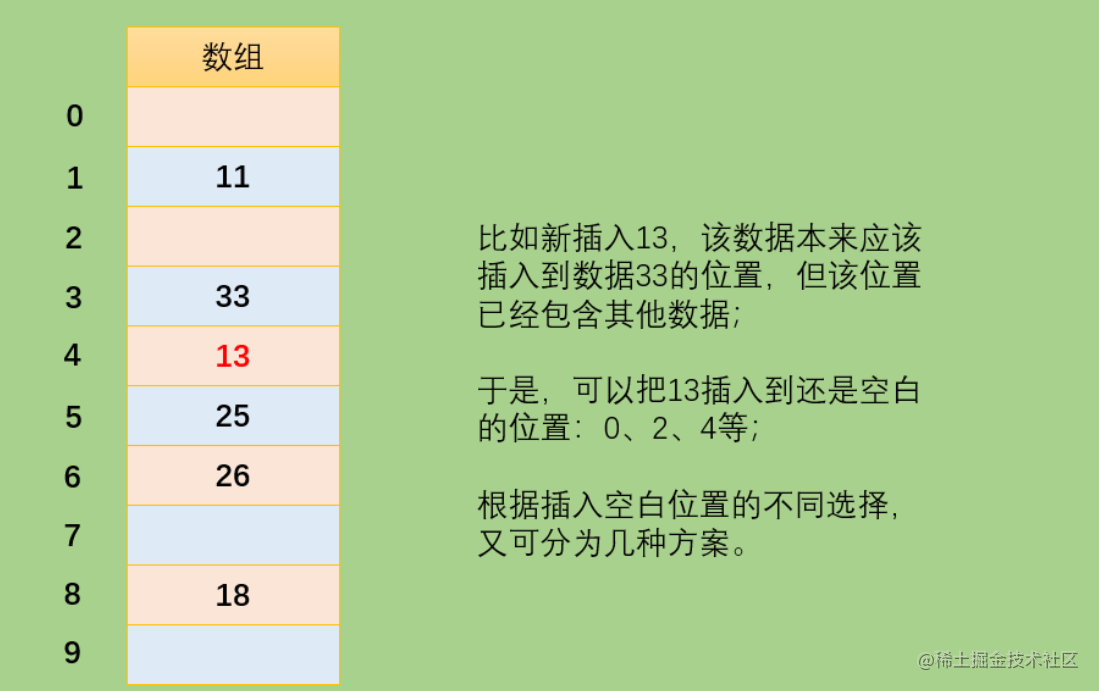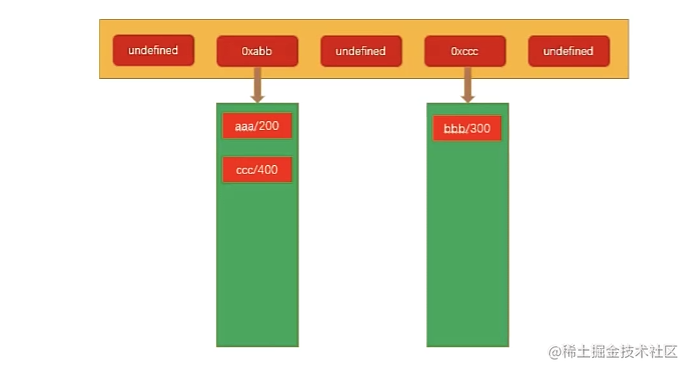2023-02-0500

## 目录

1. 哈希表介绍和特性
2. 哈希表的一些概念
3. 地址冲突解决方案
3.1 方案一：链地址法
3.2 方案二：开放地址法
4. 哈希函数代码实现
5. 哈希表封装
5.1 整体框架 v1 版
5.2 添加 put 方法 v2 版
5.3 添加 get 方法 v3 版
5.4 添加 delete 方法 v4 版
6. 哈希表的自动扩容

# 1. 哈希表介绍和特性

1. 他可以提供非常快速的 插入-删除-查找 操作

2. 无论多少数据，插入和删除都接近常量的时间：即 `O(1)` 的时间复杂度

3. 哈希表的速度比 树还要快，基本可以瞬间查找到想要的元素

4. 哈希表相对于树来说编码要容易很多

PS: 树也是一种基础的数据结构，`js` 中没有树，我们下一章就会讲树

1. 哈希表中的数据没有顺序，所以不能以一种固定的方式来遍历其中的元素

2. 通常情况下，哈希表中的 `key`不允许重复的。# 2. 哈希表的一些概念

1. 哈希化：大数字转化成数组范围内下标的过程，称之为哈希化
2. 哈希函数：我们通常会将单词转化成大数字，把大数字进行哈希化的代码实现放在一个函数中，该函数就称为哈希函数
3. 哈希表：对最终数据插入的数组进行整个结构的封装，得到的就是哈希表

• 哈希化过后的下标依然可能重复，如何解决这个问题呢？这种情况称为冲突，冲突是不可避免的，我们只能解决冲突

# 3. 地址冲突解决方案

## 3.1 方案一：链地址法

1. 链地址法解决冲突的办法是每个数组单元中存储的不再是单个数据，而是一个链条
2. 这个链条使用什么数据结构呢？常见的是数组或者链条
3. 比如链表，也就是每个数组单元中存储着一个链表。一旦发现重复，将重复的元素插入到链表的首端或者末端即可。
4. 当查询时，先根据哈希化后的下标值找到对应的位置，再取出链表，依次查询要寻找的数据1. 数组或者链表在这其实都可以，效率上也差不多
2. 因为根据哈希化的 `index` 找出这个数组或者链表时，通常就会使用线性查找，这个时候数组和链表的效率是差不多的。
3. 当然在某些实现中，会将新插入的数据放在数组或者链表的最前面，因为觉得新插入的数据用于取出的可能性更大
4. 这种情况最好采用链表，因为数组在首位插入数据是需要所有其他项后移的，链表就没有这样的问题

## 3.2 方案二：开放地址法1. 线性探测：如果发现要插入的位置已经被占据，则 `+1`，直到探测到空白位置
2. 二次探测：二次探测就是在线性探测的基础上把步长修改了（`+1 +4 +9`
3. 再哈希法：如果发现要插入的位置已经被占据，通过算法将这个位置再次哈希化，直到得到新的位置没被占据

# 4. 哈希函数代码实现

• 哈希表的主要优点是它的速度，所以在速度上不能满足，那么就打不到设计的目的了。
• 提高速度的一个办法就是让哈希函数中尽量少的有乘法和除法。因为它们的性能是比较低的。

1. 快速的计算（霍纳法则
2. 均匀的分布

```ts```/**
* 哈希函数，将 key 映射成 index
* @param key 要转换的 key
* @param max 数组的长度（最大的数值）
* @returns
*/
function hashFunc(key: string, max: number): number {
// 1. 计算 hashCode cats => 60337 （27为底的时候）
let hashCode = 0;
const length = key.length;
for (let i = 0; i < length; i++) {
// 霍纳法则计算 hashCode
hashCode = 31 * hashCode + key.charCodeAt(i);
}

// 2. 求出索引值
const index = hashCode % max;
return index;
}
``````

# 5. 哈希表封装

## 5.1 整体框架 v1 版

```ts```class HashTable<T = any> {
// 创建一个数组，用来存放链地址法中的链
storage: [string, T][][] = [];
// 定义数组的长度
private length: number = 7;
// 记录已经存放元素的个数
private count: number = 0;
}
``````

1. `storage`：创建一个数组，用来存放链地址法中的链
2. `length`：定义数组的长度
3. `count`：记录已经存放元素的个数

## 5.2 添加 put 方法 v2 版

`put` 方法的作用是插入&修改数据

• 因为，当使用者传入一个`<key,value>`
• 如果原来不存在该 `key`，那么就是插入操作
• 如果已经存在该 `key`，那么就是修改操作
```ts```put(key: string, value: T) {
// 1.根据key 获取数组中对应的索引值
const index = this.hashFunc(key, this.length);

// 2. 取出索引值对应位置的数组
let bucket = this.storage[index];

// 3. 判断 bucket 是否有值
if (!bucket) {
bucket = [];
this.storage[index] = bucket;
}

// 4. 确定已经有一个数组了，但是数组中是否已经存在 key 时不确定的
let isUpdate = false;
for (let i = 0; i < bucket.length; i++) {
const tuple = bucket[i];
const tupleKey = tuple;
if (tupleKey === key) {
// 修改/更新的操作
tuple = value;
isUpdate = true;
}
}

// 5. 如果上面的代码没有进行覆盖，那么在该位置进行添加
if (!isUpdate) {
bucket.push([key, value]);
this.count++
}
}
``````

```ts```const hashTable = new HashTable();

hashTable.put("aaa", 200);
hashTable.put("bbb", 300);
hashTable.put("ccc", 400);
``````## 5.3 添加 get 方法 v3 版

`get` 方法的作用是根据 `key` 获取对应的值

```ts```  get(key: string): T | undefined {
// 1. 根据 key 获取索引值 index
const index = this.hashFunc(key, this.length);

// 2. 获取 bucket（桶）
const bucket = this.storage[index];
if (!bucket) return undefined;

// 3. 对 bucket 进行遍历
for (let i = 0; i < bucket.length; i++) {
const tuple = bucket[i];
const tupleKey = tuple;
const tupleValue = tuple;
if (tupleKey === key) {
return tupleValue;
}
}
return undefined;
}
``````

```ts```  get(key: string): T | undefined {
// 1. 根据 key 获取索引值 index
const index = this.hashFunc(key, this.length);

// 2. 获取 bucket（桶）
const bucket = this.storage[index];
if (!bucket) return undefined;

// 3. 对 bucket 进行遍历
for (let i = 0; i < bucket.length; i++) {
const tuple = bucket[i];
const tupleKey = tuple;
const tupleValue = tuple;
if (tupleKey === key) {
return tupleValue;
}
}
return undefined;
}
``````

## 5.4 添加 delete 方法 v4 版

`delete` 方法的作用是根据 `key` 删除对应的值

```ts```  delete(key: string): T | undefined {
// 1. 获取索引值的位置
const index = this.hashFunc(key, this.length);

// 2. 获取 bucket（桶）
const bucket = this.storage[index];
if (!bucket) return undefined;

// 3. 对 bucket 进行遍历
for (let i = 0; i < bucket.length; i++) {
const tuple = bucket[i];
const tupleKey = tuple;
const tupleValue = tuple;
if (tupleKey === key) {
bucket.splice(i, 1);
this.count--;
return tupleValue;
}
}
return undefined;
}
``````

```ts```const hashTable = new HashTable();

hashTable.put("aaa", 200);
hashTable.put("bbb", 300);
hashTable.put("ccc", 400);
hashTable.delete('aaa')
console.log(hashTable.get("aaa")); // undefined
console.log(hashTable.get("bbb")); // 300
console.log(hashTable.get("ccc")); // 400
``````

# 6. 哈希表的自动扩容

`loadFactor` 译为装载因子。装载因子用来衡量 `HashMap` 满的程度

1. 调整代码，添加 `resize` 方法

```ts```private resize(newLength) {
// 设置新的长度
this.length = newLength;

// 获取原来所有的数据，并且重新放入到新的容量数组中
// 1. 对数据进行的初始化操作
const oldStorage = this.storage;
this.storage = [];
this.count = 0;

// 2. 获取原来数据，放入新的数组中
oldStorage.forEach((bucket) => {
if (!bucket) return;

for (let i = 0; i < bucket.length; i++) {
const tuple = bucket[i];
this.put(tuple, tuple);
}
});
}
``````

1. `loadFactor` 大于 `0.75` 时，对哈希表进行两倍的扩容
2. `loadFactor` 小于 `0.25` 时，对哈希表进行两倍的缩容

2. 修改 `put` 方法

```diff```  put(key: string, value: T) {
// 1.根据key 获取数组中对应的索引值
const index = this.hashFunc(key, this.length);
console.log({ index });
// 2. 取出索引值对应位置的数组
let bucket = this.storage[index];

// 3. 判断 bucket 是否有值
if (!bucket) {
bucket = [];
this.storage[index] = bucket;
}

// 4. 确定已经有一个数组了，但是数组中是否已经存在 key 时不确定的
let isUpdate = false;
for (let i = 0; i < bucket.length; i++) {
const tuple = bucket[i];
const tupleKey = tuple;
if (tupleKey === key) {
// 修改/更新的操作
tuple = value;
isUpdate = true;
}
}

// 5. 如果上面的代码没有进行覆盖，那么在该位置进行添加
if (!isUpdate) {
bucket.push([key, value]);
this.count++;

+     // 发现 loadFactor 比例已经大于 0.75，那么就直接扩容
+     const loadFactor = this.count / this.length;
+     if (loadFactor > 0.75) {
+       this.resize(this.length * 2);
+     }
}
}
``````

3. 修改 `delete` 方法

```diff```  delete(key: string): T | undefined {
// 1. 获取索引值的位置
const index = this.hashFunc(key, this.length);

// 2. 获取 bucket（桶）
const bucket = this.storage[index];
if (!bucket) return undefined;

// 3. 对 bucket 进行遍历
for (let i = 0; i < bucket.length; i++) {
const tuple = bucket[i];
const tupleKey = tuple;
const tupleValue = tuple;
if (tupleKey === key) {
bucket.splice(i, 1);
this.count--;

+       // 发现 loadFactor 比例已经小于 0.75，那么就直接缩容
+       const loadFactor = this.count / this.length;
+       if (loadFactor < 0.25 && this.length > 7) {
+         this.resize(Math.floor(this.length / 2));
+       }

return tupleValue;
}
}
return undefined;
}
``````

```ts```const hashTable = new HashTable();

hashTable.put("aaa", 200);
hashTable.put("bbb", 300);
hashTable.put("ccc", 400);
hashTable.put("ddd", 500);
hashTable.put("eee", 600);
console.log(hashTable.storage.length); // 7
hashTable.put("fff", 600);
console.log(hashTable.storage.length); // 14
hashTable.delete("fff");
hashTable.delete("eee");
console.log(hashTable.storage.length); // 14
hashTable.delete("ddd");
console.log(hashTable.storage.length); // 7
``````